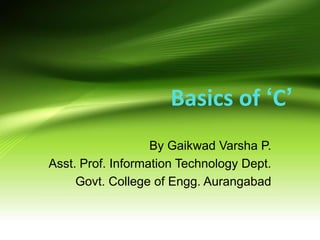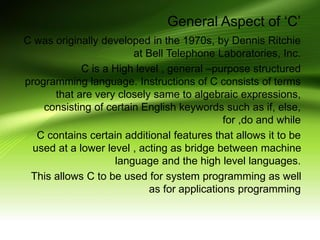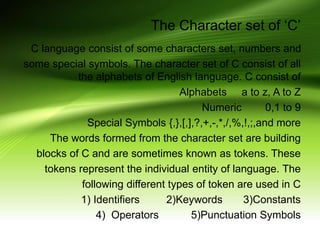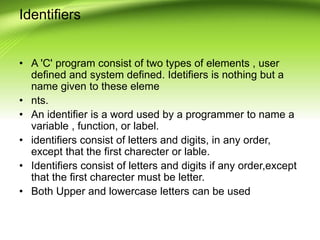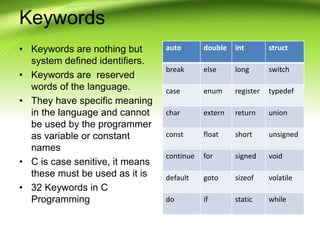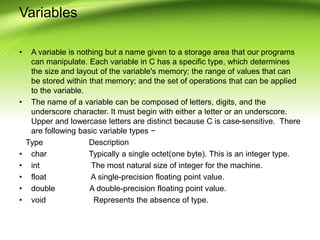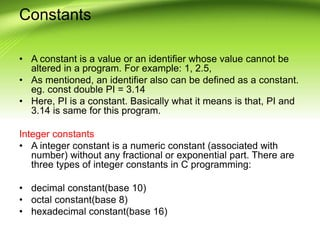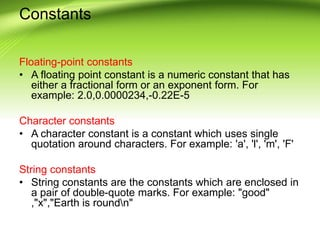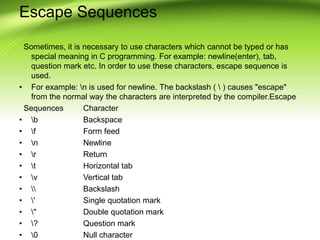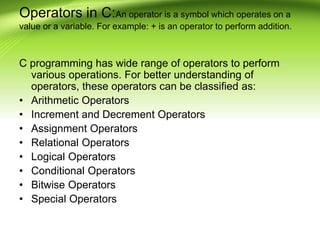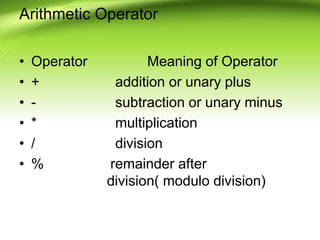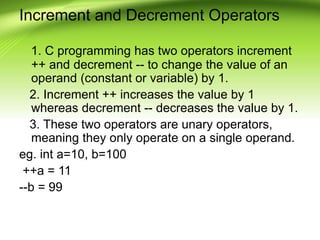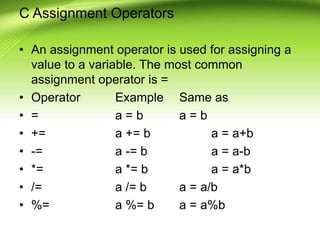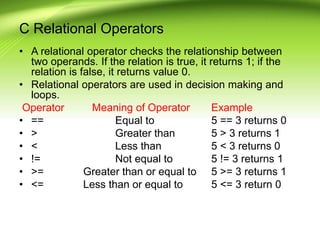1 de 14

### Basics of C.ppt

1. Basics of ‘C’ By Gaikwad Varsha P. Asst. Prof. Information Technology Dept. Govt. College of Engg. Aurangabad
2. General Aspect of ‘C’ C was originally developed in the 1970s, by Dennis Ritchie at Bell Telephone Laboratories, Inc. C is a High level , general –purpose structured programming language. Instructions of C consists of terms that are very closely same to algebraic expressions, consisting of certain English keywords such as if, else, for ,do and while C contains certain additional features that allows it to be used at a lower level , acting as bridge between machine language and the high level languages. This allows C to be used for system programming as well as for applications programming
3. The Character set of ‘C’ C language consist of some characters set, numbers and some special symbols. The character set of C consist of all the alphabets of English language. C consist of Alphabets a to z, A to Z Numeric 0,1 to 9 Special Symbols {,},[,],?,+,-,*,/,%,!,;,and more The words formed from the character set are building blocks of C and are sometimes known as tokens. These tokens represent the individual entity of language. The following different types of token are used in C 1) Identifiers 2)Keywords 3)Constants 4) Operators 5)Punctuation Symbols
4. Identifiers • A 'C' program consist of two types of elements , user defined and system defined. Idetifiers is nothing but a name given to these eleme • nts. • An identifier is a word used by a programmer to name a variable , function, or label. • identifiers consist of letters and digits, in any order, except that the first charecter or lable. • Identifiers consist of letters and digits if any order,except that the first charecter must be letter. • Both Upper and lowercase letters can be used
5. Keywords • Keywords are nothing but system defined identifiers. • Keywords are reserved words of the language. • They have specific meaning in the language and cannot be used by the programmer as variable or constant names • C is case senitive, it means these must be used as it is • 32 Keywords in C Programming auto double int struct break else long switch case enum register typedef char extern return union const float short unsigned continue for signed void default goto sizeof volatile do if static while
6. Variables • A variable is nothing but a name given to a storage area that our programs can manipulate. Each variable in C has a specific type, which determines the size and layout of the variable's memory; the range of values that can be stored within that memory; and the set of operations that can be applied to the variable. • The name of a variable can be composed of letters, digits, and the underscore character. It must begin with either a letter or an underscore. Upper and lowercase letters are distinct because C is case-sensitive. There are following basic variable types − Type Description • char Typically a single octet(one byte). This is an integer type. • int The most natural size of integer for the machine. • float A single-precision floating point value. • double A double-precision floating point value. • void Represents the absence of type.
7. Constants • A constant is a value or an identifier whose value cannot be altered in a program. For example: 1, 2.5, • As mentioned, an identifier also can be defined as a constant. eg. const double PI = 3.14 • Here, PI is a constant. Basically what it means is that, PI and 3.14 is same for this program. Integer constants • A integer constant is a numeric constant (associated with number) without any fractional or exponential part. There are three types of integer constants in C programming: • decimal constant(base 10) • octal constant(base 8) • hexadecimal constant(base 16)
8. Constants Floating-point constants • A floating point constant is a numeric constant that has either a fractional form or an exponent form. For example: 2.0,0.0000234,-0.22E-5 Character constants • A character constant is a constant which uses single quotation around characters. For example: 'a', 'l', 'm', 'F' String constants • String constants are the constants which are enclosed in a pair of double-quote marks. For example: "good" ,"x","Earth is roundn"
9. Escape Sequences Sometimes, it is necessary to use characters which cannot be typed or has special meaning in C programming. For example: newline(enter), tab, question mark etc. In order to use these characters, escape sequence is used. • For example: n is used for newline. The backslash ( ) causes "escape" from the normal way the characters are interpreted by the compiler.Escape Sequences Character • b Backspace • f Form feed • n Newline • r Return • t Horizontal tab • v Vertical tab • Backslash • ' Single quotation mark • " Double quotation mark • ? Question mark • 0 Null character
10. Operators in C:An operator is a symbol which operates on a value or a variable. For example: + is an operator to perform addition. C programming has wide range of operators to perform various operations. For better understanding of operators, these operators can be classified as: • Arithmetic Operators • Increment and Decrement Operators • Assignment Operators • Relational Operators • Logical Operators • Conditional Operators • Bitwise Operators • Special Operators
11. Arithmetic Operator • Operator Meaning of Operator • + addition or unary plus • - subtraction or unary minus • * multiplication • / division • % remainder after division( modulo division)
12. Increment and Decrement Operators 1. C programming has two operators increment ++ and decrement -- to change the value of an operand (constant or variable) by 1. 2. Increment ++ increases the value by 1 whereas decrement -- decreases the value by 1. 3. These two operators are unary operators, meaning they only operate on a single operand. eg. int a=10, b=100 ++a = 11 --b = 99
13. C Assignment Operators • An assignment operator is used for assigning a value to a variable. The most common assignment operator is = • Operator Example Same as • = a = b a = b • += a += b a = a+b • -= a -= b a = a-b • *= a *= b a = a*b • /= a /= b a = a/b • %= a %= b a = a%b
14. C Relational Operators • A relational operator checks the relationship between two operands. If the relation is true, it returns 1; if the relation is false, it returns value 0. • Relational operators are used in decision making and loops. Operator Meaning of Operator Example • == Equal to 5 == 3 returns 0 • > Greater than 5 > 3 returns 1 • < Less than 5 < 3 returns 0 • != Not equal to 5 != 3 returns 1 • >= Greater than or equal to 5 >= 3 returns 1 • <= Less than or equal to 5 <= 3 return 0